# Closed Organ Pipe

The organ pipe in which one end is opened and another end is closed is called organ pipe. Bottle, whistle, etc. are examples of closed organ pipe.

The different mode of the vibration in the closed organ pipe is discussed as below.

1. First mode of vibration [N (a)]
In the first mode of vibration in the closed organ pipe, an antinode is formed at the open end and a node is formed at the close end. If ‘l’ be the length of pipe and be the wavelength of wave emitted in this mode of vibration.
Then,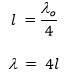if ‘μ’ be the velocity of sound and to be the frequency of wave in this mode of vibration.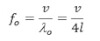This is fundamental frequency of 1st harmonics.

2. Second mode of vibration:

In this mode of vibration two antinodes and two nodes are formed as in fig. If ‘λ’ be the length of pipe and be the wavelength of wave emitted in this mode of vibration. Then,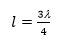f μ be the velocity of wave and f1 be the frequency of wave, in this mode of vibration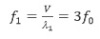This is the frequency of 1st overtone of third harmonics.

3. Third mode of vibration:
In this mode of vibration three antinodes and three nodes are formed as in fig. If ‘l’ be the length of the pipe and be the wavelength of wave admitted in this mode of vibration. Then,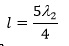ff μ be the velocity of wave and f2 be the frequency of wave. If this mode of vibration,
f2 = 5f0
This is the frequency of 2nd overtone & firth harmonics.
In this way, for the 4th, 5th…. modes of vibration the frequency of wave emitted are 7f0, 9f0……. which are called 7th , 9th …… harmonics i.e. in the closed organ pipe only odd harmonic are present.